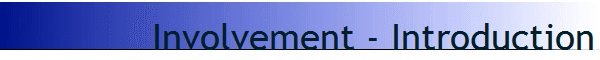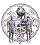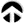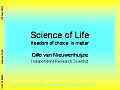## The mathematics of involvementIntroduction

The following is a expose on various ideas which are related to the mathematics of involvement.

All of them are pertinent to the way in which scientists have created a model of reality which is in fact based on a linguistic model only.
What mathematicians and scientists call "real" is in fact only a linguistic construct; it has no reality per se.
The whole basis of this belief and this mis-understanding of reality is tied in with the belief in the written word: the book-based belief systems.
In that sense science has become a religion like any other book-based belief, and needs to be regarded as such.

It is very important to realise that the reality of science is not real (it is a belief); and that the scientific 'object' is only/merely a linguistic object; a construct of words.

In order to bring the ideas of The Mathematics of Involvement together it is necessary to introduce a couple of notions which are unfamiliar to most people.
I had to create these concepts in order to come to understand and to be able to analyse the problems that we are facing in science, which are caused only by the fact that science has been turned into a belief system in which the understanding of the origin of the beliefs and cause for that belief are no longer scrutinised.

At this point I want to give a brief introduction to some of the notions tied in with this study into the linguistic nature of science, because it is at that level that we have to resolve the problems with at present we experience in science.
In this page i will only introduce these notions with a brief explanation, to be able to extend those concepts later (follow the links in the text).

### Maturana & Varela

One of the ideas which is relevant in this case is the notion of "languiging" as introduced by Maturana and Varela.
For them, the notion of language as an object was as unrealistic as the notion of reality was for David Bohm.
It is evidence that we create our (collecxtive/common) understanding by the use of language (because we communicate it amongst each other).
Language is an instrument, and as any scientific instrument it must be researched and calibrated.

### Referon Analysis

An extension of this notion of languaging, is the concept which I introduced many of years ago, called "Referon Analysis".
It is the analysis of (scientific/physical/mathematical/logical) reference systems.
This based on the concept of mathematics were in the description of any mathematical exploration and calculation, is always necessary to introduce the explicit description of the foundation of the reference system that is being used.
In mathematics that will take the form of the axial set (for example: the XYZ axis used as the basis for mathematical operations/calculations in physics).

### Langhaar

We must also understand this in terms of dimensional analysis (a very closely related concept), because in dimensional analysis it is clear that whatever we conceive (as a mental object) is determined by our definition of that formulation.
In dimensional analysis it is clear that there is a limitation/restriction on the validity of whatever we describe, specifically by the relationships between the parameters that we use in our discussion/description/formulation/equation.
Dimensional-analysis has a mathematical operation known as the calculation of the dimensionless parameter in which a limiting formulation for the viability of that equation can be obtained.
It is simple mathematical operation which however formulates how the perspective of the Observer will have its boundaries its limitations and these are based only on the way the scientist includes or excludes parameters in the consideration.

### The Collapse of the Vector of State

This is a related to a notion of quantum theory known as the "collapse of the vector of state".
This notion addresses the finding that reality must, by definition, be a realisation, because whatever we look at in the universe must be based on the same principles of the formation of matter, out of molecules, out of the interaction of atoms; as is the case for our own body and thus for our own existence.
That means that, whatever we perceive, it will always be the result of an interference pattern (an interference field) between our own being and the being of the universe.

### Gabor Holograms

At that level we need the understanding of (for example) the hologram theory of Gabor, or (in more general terms) the concept of field interference (a wave interference system),  where it is possible to make clear where wave amplification or wave suppression by the superposition of states.
It is in that sense that the collapse of the vector of state describes how our observation is based on the principle of how we observe.

### Uri Fidelman

Uri Fidelman has made that much more explicit in his way of looking at the findings of cognitive science.
As he pointed out, in the research studies of scientists, their theories are always produced by their use of their mind, thus their brain, thus their body.
in fact, he found that the equations of Einstein (known as E=MC2) are ‘the same’ as those of Newton (known as F=m.a) or the equations of Ohm (known as I=O2.R).
As he points out, the formulation of the equation is based on the way our brain integrates information.
That is a law of cognition, and thus a law of cognitive science and NOT of physics.
In other words: the so-called laws of physics are always laws of the mind.

### Grinder & Bandler

At that level it is possible to put a link to another consideration, which is that of neuro-linguistic programming; better known by its abbreviation of NLP.
This was created by in the interplay of a mathematician and linguist who were looking for the way people create models of their actions.
In researching various very effective therapists, they discovered that the explanatory model that those practitioners used, were different from what they were actually doing.
So even though there was a rationalisation for what these people are doing, to explain the effectively of whatever therapy they applied, it turned out that their actions did NOT correspond with their theoretical explanations.
In fact, the only practitioner where theory and practice seem to coincide quite well was the work of hypnotherapist Milton Ericsson.
Again it points to a link between the way we think and the way we communicate between us which is also the basis of how we formulate our consensus in terms of the of the languaging that we use.

### van Schooneveld

Another researcher who addressed the same in a different way was Cees van Schooneveld.
He was a Slavic linguist who looked at the way the pronoun propositions of the structure of language actually relates the Observer to the Observer.
Clasical Theory always denied that this relationship existed, while Quantum Theory implied that it must be considered.
Cees van Schooneveld created an explanatory model specifying how we relate to the world around us, in a set of words of which the relationship turned out to be that of a Tesseract (a four dimensional cube).
A 4D Cube is essentially the three dimensional reference system (XYZ) plus the added potential of turning the system (cube) inside out on itself. (See also the work of Lawrence Edwards, in "The Vortex of Life".)

### von Bertalannfy

That is the essence of what we find in the description of system theory, which also has four levels of description.
In that theory, they are considered to be the levels of 1) the system state, 2)  the process, 3) the transformation and 4) the integration.
Integration again is that where the system needs to turn inside out on itself in order to be able to dynamically maintain its integration with/in its context.

### Total System Inversion

In this website, scienceoflife.nl there are many texts to explain how that systemic inversions (Total System Inversion) must be the basis for the continued integration of any system within its context.
If it cannot rotate-invert on itself (as has been described also in the book "The Vortex of Life" by Lawrence Edwards) then that system cannot reposition itself and cannot recalibrate itself in its dynamic interaction/integration with/in its environment/context.

### Mathematical Operators (mathematicians)

In System Theory these inversion-rotations are described as a system of mathematical operations.
We can connect that to the model of reference analysis in order to see that every mathematical description has 1) values, 2) has functions, 3) has operations/operators and 4) it has the equation sign.
Mathematicians tend to assume that the values are determined by the validity of the operations/operators.
They ignore the findings of the more fiundamental parts of mathematics, which is that the valus are produced by the functions which are actually created by our assumptions.
This has made explicit in system theory and dimensional analysis.

### Mathematical Signs (functions)

What is even less understood is that the same applies to the formulation of the mathematical operators (which are in fact of the logical operators), such as the mathematical signs for: +, -, ×, ÷, integration and differentiation; and all other mathematical operators.

Because the mathematical operators in fact specify how we change our relationship to whatever we observed and thereby corresponds with this four dimensional grid as described by Cees van Schooneveld.

### Equation Sign (doing Mathematics)

It means that in the mathematical operations we must look at the relationship between 1) the values, 2) the functions and 3) the operators, but at a deeper level all of it always falls in place by the use of 4) the equation sign.
In the equation sign the mathematician/physicist actually establishes the validity of the 'outcome' of the equation with respect to the 'observation' of the physicist.
That means that the equation sign with its two 'bars' separated by a spave, treüresents the boundary/interface between the observer and the observed.
The Equation Sign is actually a description of our interface with and with in our environment.

In other words the equation sign of mathematical theory has the characteristics of the Tesseract described by Cees van Schooneveld, and must comply with the mental physiological brain functions described by Uri Fidelman and also found in the studies of NLP (neuro-linguistic programming).

### Jon Cunnyngham

A way to understand this deeper understanding of mathematics can be seen in the work of econometrist Jon Cunnyngham, who combined the form of mathematics used in the calculation of weather patterns with the kind of mathematics used in psychiatry psychological studies.
in fact he made use of the mirroring capacity of the mathematical equations.
Because in mathematics (as used in physics) the equations are normally interpreted as being are predictive and thus reproductive and thereby representatives for the findings of the Observer are and thus typically has the form of equations like ax + by + cz = ...

However, in psychiatry and in psychology this cannot be the case, because there people have freedom of choice.
(Psychiatry/psychology does not account for this in a direct manner.
Instead, what is looked at is the multiplicity of actions/behaviour/mentality: regarded as internal split states.
In fact, they are inconsistent internal states due to lack of coherence between different choices. In Freedom of Choice calculations they are seen as emergent rifts in the interfacing dynamic, due to the specific choices that were made.)

### Parameters vs Variables

Thus psychiatrists/prychologists consider the different/split/double/multiple mental states of the people they study.
They try to model these multiplicities by using the same exuations ad described for modelling the behaviour of other people.
But in this case they redo the calculations, altering/adapting the standard parameters of the equations, to see if they can obtain a better description.
Therein the so-called Operators in the equations are not specifiable, and need to be (re)defined by interpretation.
In mathematics this is known as a standard weighing/smoothing/fitting/shooting technique.

### Dual Computation

How this works is best seen in the example of equations of the type ax + by + cz =....
It is possible to regard, as is usual, that the variables are x, y and z.
From the perspective of mathematics it is equally possible to 'solve the equation' for a, b and c.
That means that the role/function of a,b,c and x,y,z are interchanged; the variables become the parameters and vice versa.

Once the relationship between the variables X,Y,Z is determined on the basis of references system as a defined in Referon Analysis (and Dimensional Analysis) then it must be clear that a change of values of outcome must be determined by a change of the parameters A,B,C in the equation.

### River & Bedding

An example in physics where a similar ‘swap’ is done, is in the calculation of the interplay between a river and the bedding.
For one step of the calculation, the bedding is regarded as if ‘fixed’, and the stream pattern of the flow of the river is computed.
In the next step the flow pattern of the river is assumed to be a given, and the effect on the reshaping of the river bedding is computed.
In that way, ‘stepping on both sides of the boundary’, the calculation is done until the outcome of the computation matches the findings in nature.

### State vs Phase

For that reason, the approach of Jon Cunningham was to do a double calculation (in the same way as can be done in system theory) in which on the one hand the values of X,Y,Z will be determined out of the established parameter values as would normally be considered; on the other hand A,B,C can be calculated in a a same manner (as 'variables') by assuming the calculated values of X,Y,Z as (momentarily) given.

There are some other concept which will need to be introduced for the mathematics of involvement, which will at that time be added to this description.

- - -

Let me summarise the points which I have made.

• mathematics it is often presented as a descriptive technique by which we attribut values to variations of observations.
• every mathematical model is a mental construct/code/communication/language
• every mathematical model is a linguistic construct, and must always comply with the rules for languaging
• Maturana & Varela, Grinder & Bandler, van Schooneveld and others describe those constraints
• Mental elemental principles are functions of our mind, as described by Uri Fidelman
• Mental elemental principles are functions of our body, as described by Verveen and Selye.
• The mathematical relationship is a reflection of our relationship with/in context
• The mental formulations must reflect our bodily relationships to ensure maintained health
• We can correlate computations of physics with psychological calculations, as described by Jon Cunnyngham
• 'correct outcome of calculation' is ALWAYS based on the match between the model and our expectation.
• The measurement results never matter; only the (effect of the) decisions we take matter.
• WE NEED A MATHEMATICS OF INVOLVEMENT to calibrate the matching criteria/processes/outcome.

The mathematics of involvement must describe 1) the observation (verb), 2) the observing (noun), 3) the observer, and 4) the relationship/interity within context, as experienced in our living body.[Welcome] [Core Concepts] [Topics] [Participants] [Publications] [Research] [Projects]